# JEE Main 2020 Maths Paper With Solutions Sep 2 Shift 1

JEE Main 2020 Maths paper September 2 Shift 1 solutions are available on this page. These solutions are given in a step by step manner so that students can easily understand the problems. Students are recommended to revise and learn these so that they can score higher ranks for the JEE Main exam. The solutions are also available in PDF format for free.

### September 2 Shift 1 - Maths

Question 1.A line parallel to the straight line 2x-y = 0 is tangent to the hyperbola (x2/4)-(y2/2) = 1 at the point (x1, y1). Then x12+5y12 is equal to:

1. a) 6
2. b) 10
3. c) 8
4. d) 5

Solution:

1. T: (xx1/4)-(yy1/2) = 1..(1)

t: 2x-y = 0 is parallel to T

T: 2x-y = λ ..(2)

Now compare (1) and (2)

x1 ÷(4/2) = y1 ÷(2/1) = 1/λ

x1 = 8/λ and y1 = 2/λ

(x1, y1) lies on hyperbola

(64/4λ2) -(4/2λ2) = 1

14 = λ2

Now x12+5y12 = (64/λ2) +5(4/λ2)

= 84/14

= 6

Question 2. The domain of the function f(x) = sin-1 [(|x|+5)/(x2+1)] is (-∞, -a]U[a,∞). Then a is equal to:

1. a) (√17-1)/2
2. b) √17/2
3. c) (1+√17)/2
4. d) (√17/2)+1

Solution:

1. -1≤ (|x|+5)/x2+1 ≤1

-x2-1≤|x|+5≤ x2+1

Case 1

-x2-1≤ |x|+5

This inequality is always right for all x∈R.

Case 2:

|x|+5 ≤ x2+1

x2-|x| ≥4

x2-x-4 ≥0

(|x|-(1+√17)/2)(|x|-(1-√17)/2) ≥0

|x|≤(1-√17/2) (Not possible)

Or |x| ≥ (1+√17/2)

x∈(-∞, (-1-√17)/2] U[(1+√17)/2, )

a = (1+√17)/2

Question 3. If a function f(x) defined by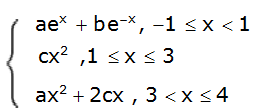be continuous for some a, b, c ∈ R and f’(0)+f’(2) = e, then the value of a is:

1. a) 1/(e2-3e+13)
2. b) e/(e2-3e-13)
3. c) e/(e2+3e+13)
4. d) e/(e2-3e+13)

Solution:

1. f(x) is continuous

at x =1 At x = 1, b = ce - ae2

at x =3

9c = 9a+6c

c = 3a

Now f ’(0) +f ‘(2) = e

a-b+4c = e

a-e (3a-ae)+4×3a = e

a-3ae+ae2+12a = e

13a-3ae+ae2 = e

a = e/(13-3e+e2)

Question 4. The sum of the first three terms of a G.P. is S and their product is 27. Then all such S lie in

1. a) (-∞, -9]∪[3, ∞)
2. b) [-3, ∞)
3. c) (-∞, -9]
4. d) (-∞, -3]∪[9, ∞)

Solution:

1. (a/r).a.ar = 27

a = 3

(a/r)+a+ar = S

(1/r)+1+r = S/3

r+(1/r) = (S/3)-1

r+(1/r) ≥ 2 or r+(1/r) ≤ -2

(S/3) ≥ 3 or (S/3) ≤ -1

S≥ 9 or S ≤ -3

S∈(-∞, -3]∪[9,∞)

Question 5. If R = {(x,y):x,y ∈ Z, x2+3y2 ≤ 8} is a relation on the set of integers Z, then the domain of R-1 is:

1. a) {-1, 0, 1}
2. b) {-2, -1, 1, 2}
3. c) {0,1}
4. d) {-2,-1,0,1,2}

Solution:

1. 3y2 ≤ 8-x2

R:{(0,1), (0,-1), (1,0), (-1,0), (1,1), (1,-1), (-1,1), (-1,-1), (2,0), (-2,0), (2,1), (2,-1), (-2,1), (-2,-1)}

R: {-2, -1, 0, 1, 2} ->{-1,0,-1}

Hence R-1:{-1,0,1}-> {-2, -1, 0, 1, 2}

Question 6. The value of (1+sin (2π/9)+i cos(2π/9)/(1+sin (2π/9)-i cos(2π/9))3 is:

1. a) (-1/2)(1-i√3)
2. b) (1/2)(1-i√3)
3. c) (-1/2)(√3-i)
4. d) (1/2)(√3-i)

Solution:

1.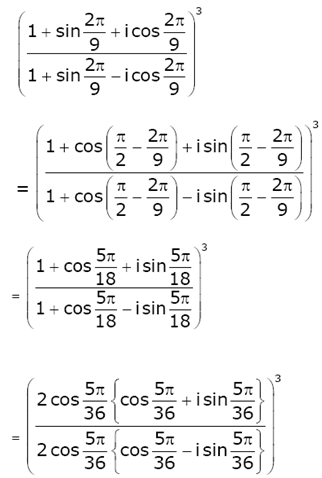= (ei(5π/36)+i(5π/36))3

= (e2i(5π/36))3

= ei(30π/36)

= ei(5π/6)

= cos (5π/6)+ i sin 5π/6

= (-√3/2)+(i/2)

Question 7. Let P(h,k) be a point on the curve y = x2+7x+2, nearest to the line, y = 3x-3. Then the equation of the normal to the curve at P is:

1. a) x+3y-62 = 0
2. b) x-3y-11 = 0
3. c) x-3y+22 = 0
4. d) x+3y+26 = 0

Solution:

1. C: y = x2+7x+2

Let P : (h, k) lies on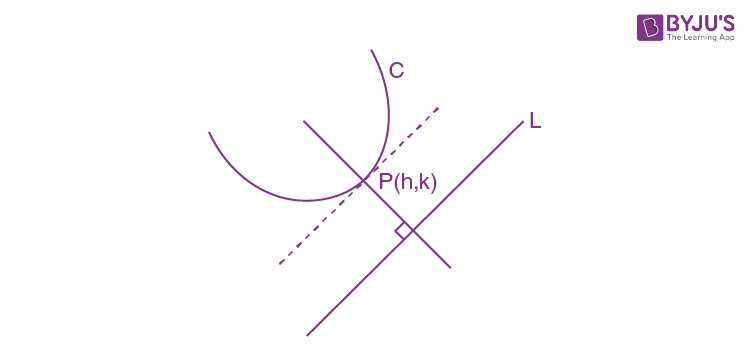Curve k = h2+7h+2 ...(1)

Now for shortest distance

Slope of tangent line at point P = slope of line L

dy/dx at P(h,k) = mL

(d/dx)(x2+7x+2) at P(h,k) = 3

(2x+7)at P(h,k) = 3

2h+7 = 3

h = -2

from (1) k = -8

P: (-2, -8)

equation of normal to the curve is perpendicular to L: 3x-y = 3

N: x+3y = λ

Pass(-2,-8)

λ = -26

N: x+3y+26 = 0

Question 8. Let A be a 2×2 real matrix with enries from {0,1} and A ≠ 0. Consider the following two statements:

(P) If A ≠I2 , then A = -1

(Q) If A =1 , then tr(A) = 2,

where I2 denotes 2×2 identity matrix and tr(A) denotes the sum of the diagonal entries of A. Then:

1. a) Both (P) and (Q) are false
2. b) (P) is true and (Q) is false
3. c) Both (P) and (Q) are true
4. d) (P) is false and (Q) is true

Solution:

1. P: A =

$$\begin{bmatrix} 1 &1 \\ 0 & 1 \end{bmatrix}$$
≠I2

And A ≠0 and A = 1 (False)

Q: A =

$$\begin{bmatrix} 1 &1 \\ 0 & 1 \end{bmatrix}=1$$

then Tr(A) = 2 (true)

Question 9. Box I contains 30 cards numbered 1 to 30 and Box II contains 20 cards numbered 31 to 50. A box is selected at random and a card is drawn from it. The number on the card is found to be a non-prime number. The probability that the card was drawn from Box I is:

1. a) 4/17
2. b) 8/17
3. c) 2/5
4. d) 2/3

Solution:

1. Let P(I) represent probability of selecting box I and P(II) represent probability of selecting box II.

P(I) = 1/2

P(II) = 1/2

In Box I, prime numbers are {2,3,5,7,11,13,17,19,23,27}

In Box II, prime numbers are {31,37,41,43,47}

Let A be event that selected number on card is non prime.

P(A) = P(I).P(A/I)+P(II).P(A/II)

= (1/2)×(20/30)+(1/2)×(15/20)

= 17/24

Now, P(I/A) = P(II).P(A/I)÷P(A)

= (1/2)×(20/30)÷ (17/24)

= (1/3)×(24/17)

= 8/17

Question 10. If p(x) be a polynomial of degree three that has a local maximum value 8 at x = 1 and a local minimum value 4 at x = 2; then p(0) is equal to :

1. a) 12
2. b) -12
3. c) -24
4. d) 6

Solution:

1. p’(1) = 0 and p’(2) = 0

p’(x) = a(x-1)(x-2)

p(x) = a[(x3/3)-(3x2/2)+2x]+b

p(1) = 8

a[(1/3)-(3/2)+2]+b = 8 ..(i)

p(2) = 4

a[(8/3)-(3×4/2)+2×2]+b = 4 ..(ii)

From equation (i) and (ii)

a = 24 and b = -12

p(0) = b = -12

Question 11.The contrapositive of the statement “If I reach the station in time, then I will catch the train” is:

1. a) If I will catch the train, then I reach the station in time.
2. b) If I do not reach the station in time, then I will catch the train.
3. c) If I do not reach the station in time, then I will not catch the train.
4. d) If I will not catch the train, then I do not reach the station in time.

Solution:

1. Statement p and q are true

Statement, then the contra positive of the implication

p->q = (~q) ->(~p)

Question 12.Let α and β be the roots of the equation, 5x2+6x-2 = 0. If Sn = αnn, n = 1,2,3,.. then:

1. a) 5S6+6S5+2S4 = 0
2. b) 6S6+5S5 = 2S4
3. c) 6S6+5S5+2S4 = 0
4. d) 5S6+6S5= 2S4

Solution:

1. 5x2+6x-2 = 0

Put x = α

2+6α-2 = 0

6α-2 = -5α2 ..(i)

Similarly

6β-2 = -5β2 ..(ii)

S6 = α66

S5 = α55

S4 = α44

Now 6S5-2S4 = 6α5+6β5-2α4-2β4

= α4(6α-2)+β4(6β-2)

= α4(-5α2)+β4(-5β2)

= -5(α66)

= -5S6

Hence 6S5+5S6= 2S4

Question 13. If the tangent to the curve y = x+sin y at a point (a,b) is parallel to the line joining (0, 3/2) and (1/2, 2), then:

1. a) b = (π/2)+a
2. b) |a+b| = 1
3. c) |b-a| = 1
4. d) b = a

Solution:

1. (dy/dx)p(a,b) = (2-3/2)/(1/2 -0)

1+cos b = 1. p: (a,b) lies on curve

cos b = 0

b = a+sin b

b = a

$$\pm$$
1

b-a =

$$\pm$$
1

|b-a| = 1

Question 14. Area (in sq. units) of the region outside (x/2)+ (y/3) = 1 and inside the ellipse (x2/4)+(y2/9) = 1 is:

1. a) 3(π-2)
2. b) 6(π-2)
3. c) 6(4-π)
4. d) 3(4-π)

Solution:

1.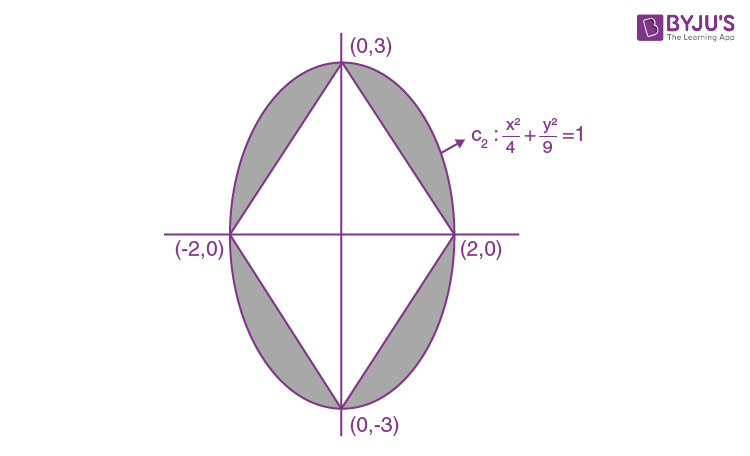Given (x/2)+ (y/3) = 1

A = 4((πab/4)-(1/2)×2×3)

= π×2×3-12

= 6(π-2)

Question 15. If |x| <1, |y| <1, and xy, then the sum to infinity of the following series - (x+y)+(x2+xy+y2)+(x3+x2y+xy2+y3)+… is:

1. a) (x+y+xy)/(1-x)(1-y)
2. b) (x+y-xy)/(1-x)(1-y)
3. c) (x+y+xy)/(1+x)(1+y)
4. d) (x+y-xy)/(1+x)(1+y)

Solution:

1. (x+y)+(x2+xy+y2)+(x3+x2y+xy2+y3)+..... ∞

= (1/x-y){(x2-y2)+(x3-y3)+(x4-y4)+.....∞

= (x2/(1-x)) -(y2/(1-y))/(x-y)

= {x2(1-y)-y2(1-x)}/(1-x)(1-y)(x-y)

= ((x+y)-xy)(x-y)/(1-x)(1-y)(x-y)

= (x+y-xy)/(1-x)(1-y)

Question 16. Let α>0, β>0, be such that α32 = 4. If the maximum value of the term independent of x in the binomial expansion of (αx1/9+βx-1/6)10 is 10k, then k is equal to:

1. a) 176
2. b) 336
3. c) 352
4. d) 84

Solution:

1. For term independent of x

Tr+1 = 10Cr(αx1/9 )10-r(βx-1/6)r

= 10Crα10-rβr.x(10-r)/9.x-r/6

Since (10-r)/9-(r/6) = 0

r = 4

T5 = 10Crα6.β4

AM≥GM

Now

$$\frac{\frac{\alpha ^{3}}{2}+\frac{\alpha ^{3}}{2}+\frac{\beta ^{2}}{2}+\frac{\beta ^{2}}{2}}{4}\geq \sqrt{\frac{\alpha ^{6}\beta ^{4}}{2^{4}}}$$

Take 4th power

(4/4)4 ≥ (α6β4/24)

α64 ≤ 24

10C4α6410C424

T510C424

T5 ≤ 10!24/(6!4!)

T5 ≤ (10×9×8×7×24)/(4×3×2×1)

Maximum value of T5 = 10×3×7×16 = 10k

k = 3×7×16

k = 336

Question 17. Let S be the set of all λ ∈ R for which the system of linear equations

2x-y+2z = 2

x-2y+λz = -4

x+λy+z=4

has no solution. Then the set S

1. a) is an empty set.
2. b) is a singleton.
3. c) contains more than two elements.
4. d) contains exactly two elements.

Solution:

1. For no solution

Δ= 0

Δ =

$$\begin{vmatrix} 2 & -1&2 \\ 1& -2 &\lambda \\ 1 & \lambda & 1 \end{vmatrix}=0$$

2(-2-λ2)+1(1-λ)+2(λ+2) = 0

-4-2λ2+1-λ+2λ+4 = 0

2-λ-1 = 0

λ= 1 or -1/2

For two values of λ, equations has no solution.

Question 18. Let X = {x∈N: 1≤x≤17} and Y = {ax+b:x∈X and a,b∈R, a>0}. If mean and variance of elements of Y are 17 and 216 respectively then a+b is equal to:

1. a) -27
2. b) 7
3. c) -7
4. d) 9

Solution:

1. X :{1,2,…17}

Y: {ax+b:x∈X and a,b∈R, a>0}

Given var(Y) = 216

(Ʃy12)/n - mean2 = 216

(Ʃy12)/17 - 289 = 216

(Ʃy12) = 8585

(a+b)2 +(2a+b)2+....+(17a+b)2 = 8585

105a2 +b2+18ab=505 ..(1)

Now Ʃy1 = 17×17

a(17×9) + 17.b = 17×17

9a+b = 17 ....(2)

from equation (1) & (2)

a = 3 and b = -10

a+b = -7

Question 19.Let y = y(x) be the solution of the differential equation, (2+sinx)/(y+1) (dy/dx) = -cos x, y>0, y(0) = 1. If y(π) = a, and (dy/dx) at x = π is b, then the ordered pair (a,b) is equal to:

1. a) (2,2/3)
2. b) (1,1)
3. c) (2,1)
4. d) (1,-1)

Solution:

1. ∫(dy/y+1) = ∫-cos x/(2+sin x)dx

ln |y+1| = -ln |2+sin| x +k

Put (0,1)

k = ln 4

Now C: (y+1)(2+sin x) = 4

y(π) = a (a+1)(2+0) = 4

⇒ a = 1

(dy/dx)x=π = b

⇒ b = -(-1)(2+0)/(1+1)

= 1

(a,b) = (1,1)

Question 20. The plane passing through the points (1,2,1), (2,1,2) and parallel to the line, 2x = 3y, z = 1 also passes through the point:

1. a) (0,-6,2)
2. b) (0,6,-2)
3. c) (-2,0,1)
4. d) (2,0,-1)

Solution:

1.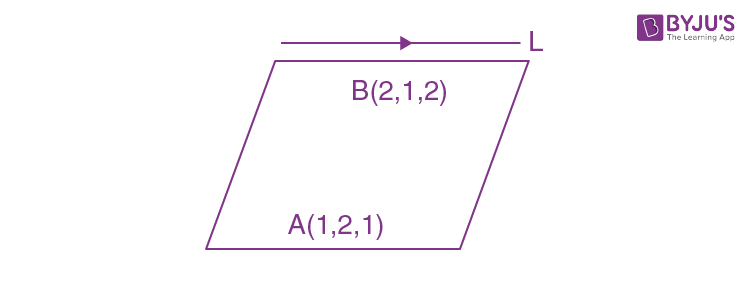Given lines are 2x = 3y, z = 1

Dr of line (3,2,0)

$$\vec{n_{p}} = \vec{AB}\times \vec{V_{L}}$$

$$\vec{n_{p}} = \left \langle 1, -1, 1 \right \rangle \times \left \langle 3,2,0 \right \rangle$$

$$\vec{n_{p}} = \left \langle -2, +3, 5 \right \rangle$$

Plane : -2(x-1)+3(y-2)+5(z -1) = 0

Plane : -2x+3y+5z+2-6-5=0

Plane : 2x-3y-5z = -9

Question 21. The number of integral values of k for which the line, 3x+4y = k intersects the circle, x2+y2-2x-4y+4 = 0 at two distinct points is…

Solution:

1.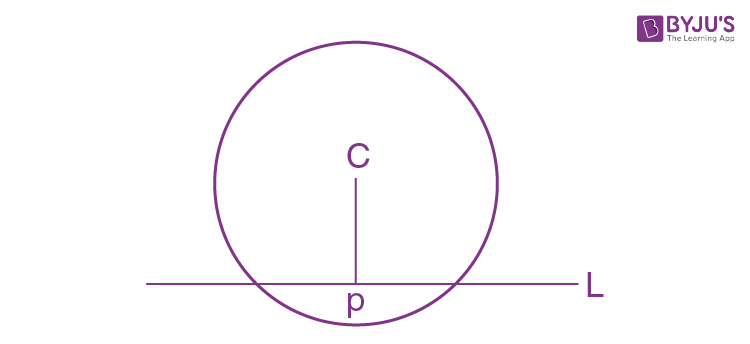c: (1,2) and r = 1

cp < r

|(3×1+4×2-k)/5| < 1

|11-k| < 5

-5<k-11<5

6<k<16

k = 7,8,9,..,15

So total 9 value of k.

Question 22.Let

$$\vec{a},\vec{b}\; and\: \vec{c}$$
be three unit vectors such that
$$\left | \vec{a}-\vec{b} \right |^{2}+\left | \vec{a}-\vec{c} \right |^{2} =8$$
. Then
$$\left | \vec{a}+2\vec{b} \right |^{2}+\left | \vec{a}+2\vec{c} \right |^{2}$$
is equal to:

Solution:

1. $$\left | \vec{a}-\vec{b} \right |^{2}+\left | \vec{a}-\vec{c} \right |^{2} =8$$
.

$$(\vec{a}-\vec{b}).(\vec{a}-\vec{b})+(\vec{a}-\vec{c}).(\vec{a}-\vec{c})=8$$

a2+b2-2a.b+a2+c2-2a.c = 8

2a2+b2+c2-2a.b -2a.c=8

a.b+a.c = -2

Now

$$\left | \vec{a}+2\vec{b} \right |^{2}+\left | \vec{a}+2\vec{c} \right |^{2}$$

= 2a2+4b2+4c2+4a.b+4a.c

= 2+4+4+4(-2)

= 2

Question 23. If the letters of the word ’MOTHER’ be permuted and all the words so formed (with or without meaning) be listed as in a dictionary, then the position of the word ’MOTHER’ is....

Solution:

1.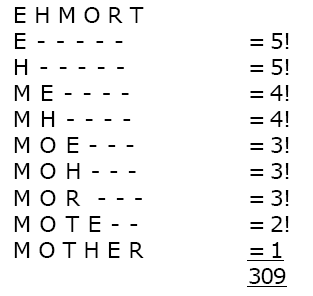Question 24.If

$$\lim_{x\to1}\frac{x+x^{2}+x^{3}+...x^{n}-n}{x-1} = 820$$
n∈N, then the value of n is equal to:

Solution:

1. Given

$$\lim_{x\to1}\frac{(x-1)}{x-1} +\frac{(x^{2}-1)}{x-1}+..\frac{(x^{n}-1)}{x-1}= 820$$

1+2+3+…+n = 820

Ʃn = 820

n(n+1)/2 = 820

n = 40

Question 25. The integral

$$\int_{0}^{2}\left | \left | x-1 \right |-x \right |dx$$
is equal to:

Solution:

1. $$\int_{0}^{2}\left | \left | x-1 \right |-x \right |dx$$

=

$$\int_{0}^{1}\left | 1-x-x \right |dx+\int_{1}^{2}\left | x-1-x \right |dx$$

=

$$\int_{0}^{1}\left | 2x-1 \right |dx+\int_{1}^{2} 1 dx$$

=

$$\int_{0}^{\frac{1}{2}}(1-2x)dx+\int_{\frac{1}{2}}^{1}(2x-1)dx+\int_{1}^{2} 1 dx$$

= [(1/2)-0-(1/4)-0]+(1-(1/4))-(1-(1/2))+1

= (1/2)-(1/4)+(3/4)-(1/2)+1

= 3/2

### JEE Main 2020 Maths Paper With Solutions Sep 2 Shift 1